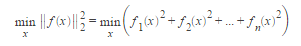Chemistry

# Nonlinear Least squares Curve fitting

There are many equations and laws in Chemistry which may be plotted in Nonlinear Least squares Curve fitting linear graphs, as well as the obvious y= nix + c, e.g. log functionsfix) = aln(x) + b; power functions f(x) = axb; exponential functions, fix) = abx; reciprocal functions, f(x) = a + blx. The transformed functions can be treated by the LLSQ LINEST approach. As we saw with the Arrhenius plot such transformations may not use the data to its best advantage. There are also many equations and laws that cannot be plotted as straight lines e.g. a double log function, f(x) = aln(x) + bln(x).

In both LibreOffice Calc and OpenOffice Calc the algorithm Solver is part of the software but in Excel you may have to install Solver for NLLSQ, see the appropriate web-site. The simplest way of showing how to use this system is using a worked example. Here use LibreOffice Calc but anyone using Excel will find similar instructions.

Solves nonlinear least-squares curve fitting problems of the formwith optional lower and upper bounds lb and ub on the components of x. x, lb, and ub can be vectors or matrices.